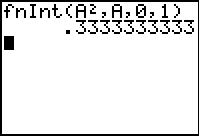# Knowledge Base

## Solution 13341: Using the fInt Function Integral Command on the TI-83 Plus Family of Graphing Calculators.

### How do I use the fInt Function Integral command on the TI-83 Plus family of graphing calculators?

The fnInt command returns the numerical integral of an expression with regards for real numbers only. The numerical integral is based on the Gauss-Kronrod method. The numerical integral is calculated with respect to the variable, given a lower limit, upper limit and a tolerance. Tolerance is optional and if not specified it is defaulted to 1E-5.

The syntax for the fnInt command is below:

fnInt(expression, variable, lower, upper[,tolerance]), between lower and upper, with specified tolerance.

Example: Find the function integral of A2, for the variable A, with 0 as the lower bound and 1 as the upper bound:

1) Press [MATH] then  to select 9:fInt(.
2) Press [ALPHA] [MATH] [x2] [,] [ALPHA] [MATH] [,]  [,]  [)] [ENTER].